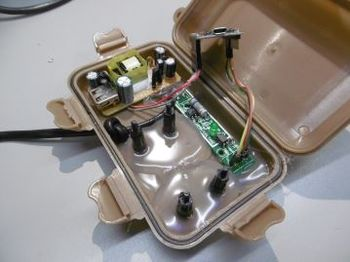# MainsFrequency2.0

Project MainsFrequency2.0A simple mains frequency counter
Status In progress
Contact bertrik
Last Update 2023-03-21

## Introduction

This project is a reboot of this earlier main frequency counter, aiming for more accuracy and lower latency.

It's based on the Arduino platform, using an ESP8266 to do the wifi/network/MQTT stuff. The frequency measurement principle is to measure the time between zero crossings (in a statistically robust way).

### Concept

Instead of just counting pulses from zero-crossings, we sample the actual 50 Hz waveform and try to estimate the zero-crossing as accurately as possible.

Desired end result:

• get more accurate frequency measurement, aiming for 1 milli-Hertz accuracy
• get more responsive frequency measurement, i.e. instantaneous value (1 second), not a running average over 50 seconds.

A suitable module for relatively safely sampling the mains voltage is this ZMPT101B module. It contains a transformer and an op-amp circuit.

Algorithm for getting accurate instantaneous frequency out of it:

• Sample the mains frequency waveform during approximately one second (say 5 - 10 kHz)
• Determine the median, lower and upper quartiles of the waveform, and shift data so its values are symmetrically around zero
• Calculate zero crossings by doing linear regression to find the zero-crossing of the wave in the region around the zero crossing (in between the quartile values), this gives sub-sample time resolution
• The linear regression runs a kind of state machine, just keeping track of sum(x), sum(y), sum(x*x), sum(x*y) is enough to perform a linear regression at any time
• Do this over the approximately 50 cycles contained in the one second data and determine average frequency

-> this should give about 1 millihertz frequency resolution in one second

## Visualisation

There are (at least) the following two ways we can output the data:

• publish frequency as a number over WiFi / MQTT for visualization as a graph-over-time on our grafana server
• idea: directly on a LED ring. The ring shows an integer number (e.g.) of 50 Hz cycles, with the color of the pixel indicating the analog value

Regarding the second idea:

• The LED ring shows the raw waveform over time, so three 50 Hz cycles show up as 3 dark spots and 3 light spots around the ring, approximately 120 degrees apart. Basically it shows the phase compared to a reference 50 Hz frequency.
• The LED ring is drawn based on a reference time (derived from the crystal oscillator), assumed to be exactly 50 Hz. A slightly fast mains waveform results in a clockwise rotation of the waveform pattern, a slightly slow mains waveform results in a counter-clockwise rotation of the waveform pattern.
• Use a colourful gradient, not just intensity.

## Hardware

For measurement with an ESP8266, like a Wemos D1 mini or nodemcu, you need to put a 180k ohm resistor in line with the output from the ZMPT101B to the A0 input. The A0 input already has a 220k/100k resistive divider, effectively becoming a 400k/100k resistive divider with the series resistor, scaling down the 0-5V range to the 0-1V range required for the ADC on the ESP8266.

The "blue pill" seems to have too low accuracy of the built-in crystal, about 100 ppm, while we need about 20 ppm to get 1 mHz resolution. Notes about blue pill crystal accuracy: https://sparklogic.ru/arduino-for-stm32/accurate-blue-pill-clock-frequency-adjustment.html

Connections
ZMPT101B Wemos D1 mini Remark
GND GND Ground
VCC 5V Powers the ZMPT101B from the wemos D1 mini
OUT A0 Analog mains waveform, 0..5V, 180 kohm resistor in series

## Software

Github project: https://github.com/bertrik/MainsFrequency

To flash the esp8266:

• install platformio
• enter the esp8266sampler directory
``` cd esp8266sampler
```
``` pio run -t upload